# High School Math : Rectangles

## Example Questions

### Example Question #11 : How To Find The Area Of A Rectangle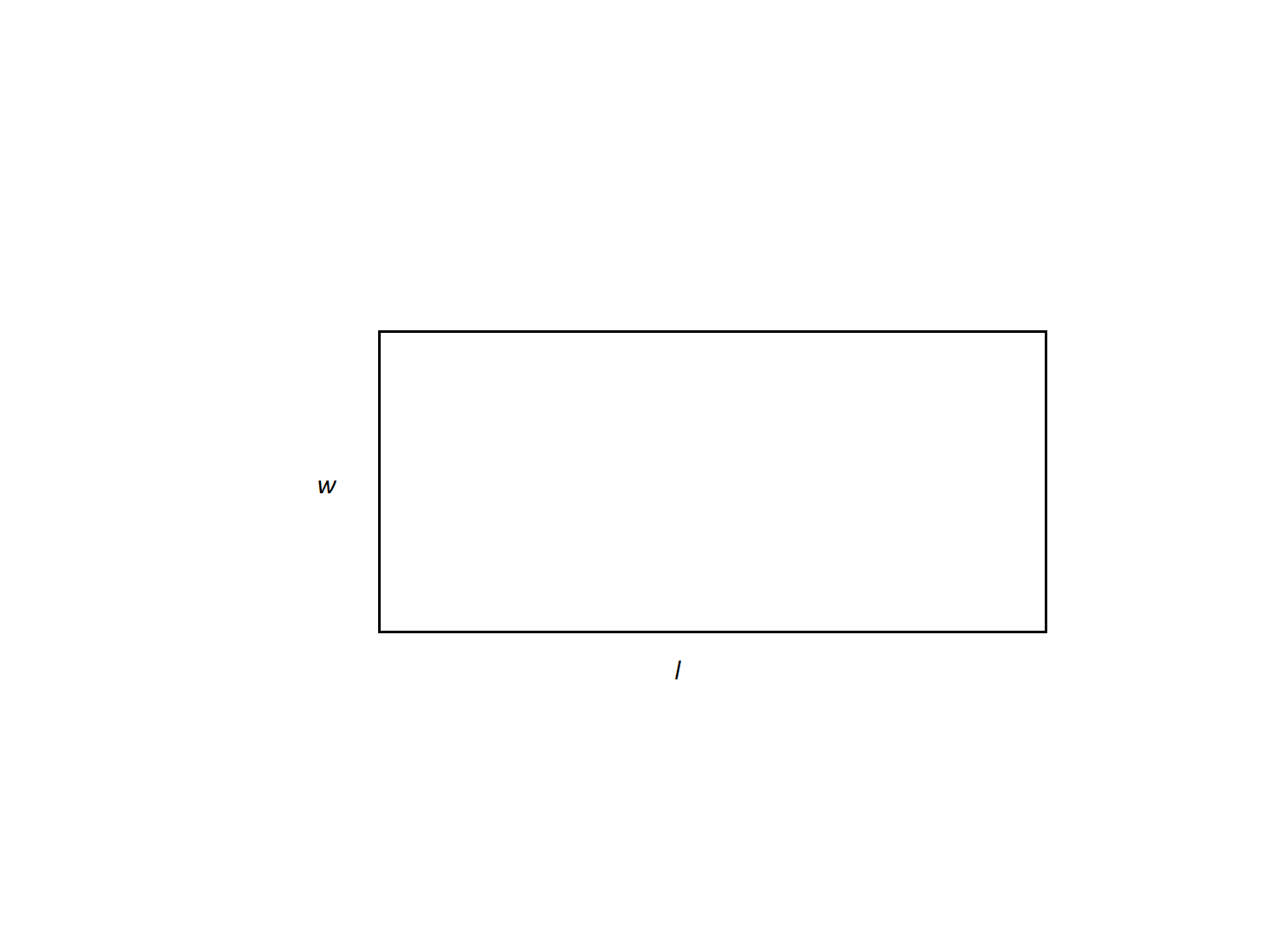Find the area of a rectangle with a length of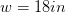and a length of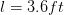.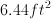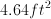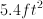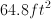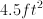Explanation:

First, we need to convert the length and width of the rectangle into similiar units.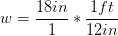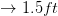Now, calculate the area.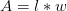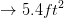### Example Question #12 : How To Find The Area Of A Rectangle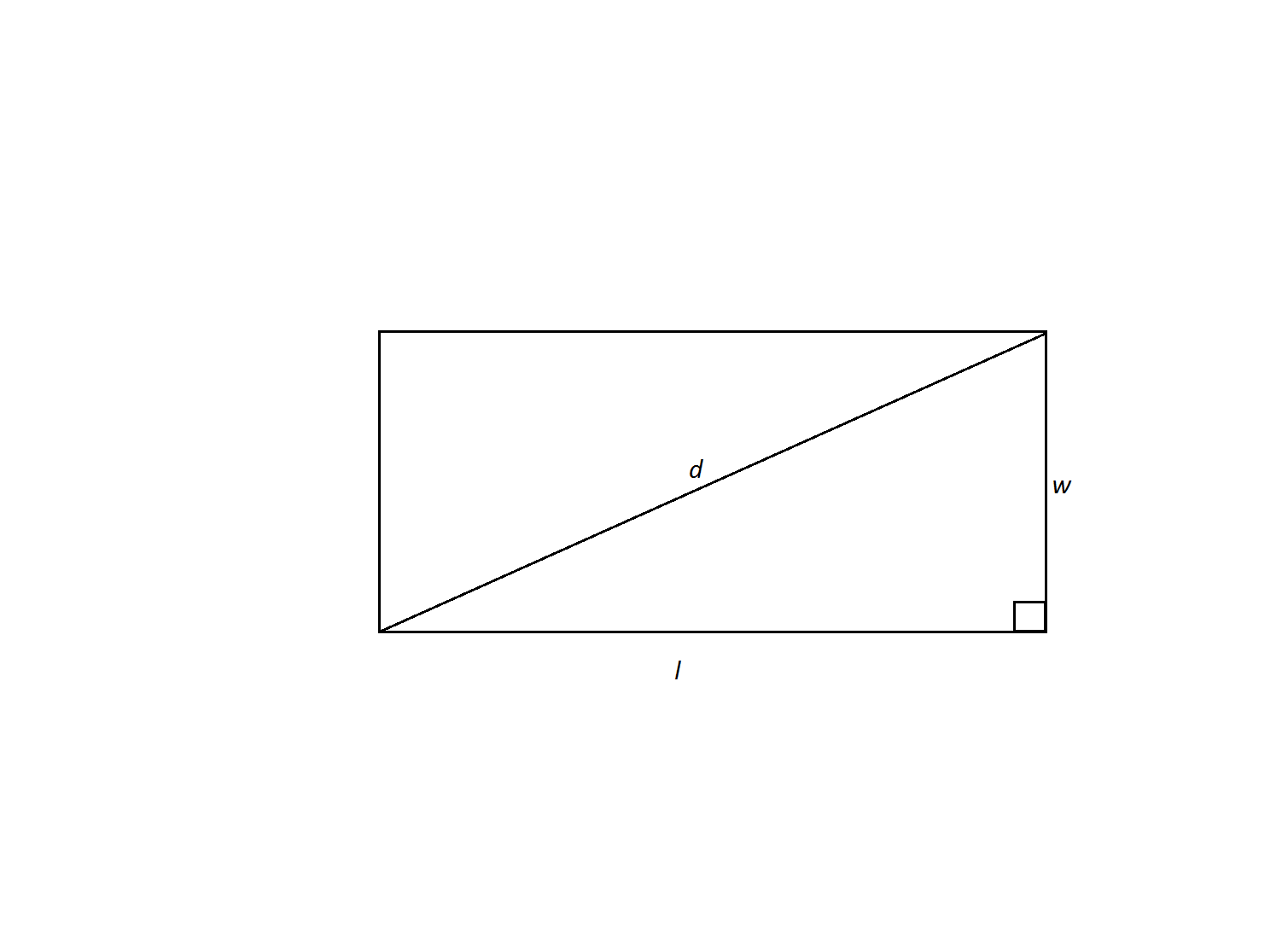A rectangle has a diagonal of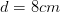and a width of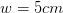. What is the area of the rectangle?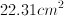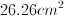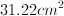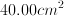Not enough information to solveExplanation:

We are given the rectangle's diagonal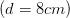and width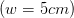and are asked to find its area. The diagonal forms two right triangles within the rectangle; therefore, we can use the Pythagorean theorem to find the length of the rectangle's missing side. Then we can use the formulato find the are of the rectangle.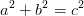In our case, we can rename the variables to match our triangle.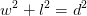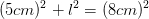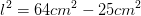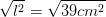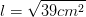Now we can calculate the area.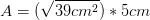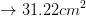### Example Question #13 : How To Find The Area Of A Rectangle

Joe has a rectangluar yard and wants to fence in his yard as well as plant grass seed.  His yard measures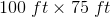.  The fence costs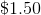per foot, and the grass seed costs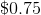per square foot.  How much money does he need to complete both projects?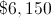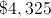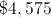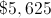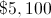Explanation:

This problem requires you to find both the perimeter (fence) and the area (grass seed) of a rectangle where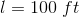and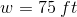.

Fence Problem (Perimeter):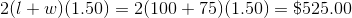Grass Seed Problem (Area):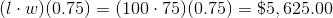So the total cost for both projects is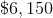.

### Example Question #14 : How To Find The Area Of A Rectangle

A rectangle has sides of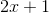units andunits. If the perimeter of the rectangle is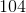units, what is its area?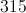units squared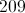units squared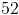units squared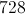units squared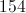units squaredunits squared

Explanation:

Since a rectangle haspairs of equal-length sides, multiplying each side byand adding the products together gives the perimeter of the rectangle. Use this fact to set up an equation with the given information about the rectangle's sides and perimeter. Solving forin this equation will provide necessary information for finding the rectangle's area: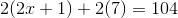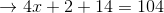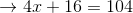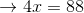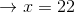Multiplying the measure of the long side of the rectangle by the measure of the short side of the rectangle gives the rectangle's area. The length of the long side is given by substituting the solution forinto the given expressionthat defines its length. The short side is, giving the following equation to calculate the area: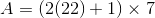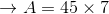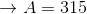units squared

### Example Question #15 : How To Find The Area Of A Rectangle

The ratio of the areas of two rectangles is. If the larger rectangle has a length ofand a width of, what is the area of the smaller rectangle?units squaredunits squaredunits squaredunits squaredunits squaredunits squared

Explanation:

The area of the larger rectangle is calculated as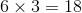units squared. Since the ratio of the larger to the smaller rectangle is, dividing the larger rectangle's area bygives the area of the smaller rectangle: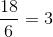units squared

### Example Question #16 : How To Find The Area Of A Rectangle

Joey is working in his yard.  In the middle of his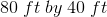rectangular yard he places a round cement fountain.  The fountain has a diameter of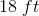.  One bag of grass seed covers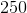square feet.  How many bags of grass seed will Joey need to cover his yard?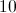Explanation:

First, find the area of the rectangular yard: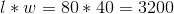square feet

Next, find the area of the round cement fountain: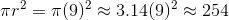square feet

Then find the difference between the two: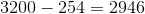square feet

Now, to get the number of grass seed bags needed, divide the area byto get approximately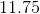bags.  Because one can't purchase a partial bag, the correct answer is the next largest whole number, orbags of grass seed.

### Example Question #4 : How To Find The Area Of A Rectangle

A rectangle has a perimeter of 40 inches.  It is 3 times as long as it is wide.  What is the area of the rectangle in square inches?

60

45

75

86

75

Explanation:

The width of the rectangle is w, therefore the length is 3w.  The perimeter, P, can then be described as P = w + w + 3w +3w

40 = 8w

w = 5

width = 5, length = 3w = 15

A = 5*15 = 75 square inches

### Example Question #5 : How To Find The Area Of A Rectangle

Angela is carpeting a rectangular conference room that measures 20 feet by 30 feet. If carpet comes in rectangular pieces that measures 5 feet by 4 feet, how many carpet pieces will she need to carpet the entire room?

30

31

600

20

29

30

Explanation:

First, we need to find the area of the room. Because the room is rectangular, we can multiply 20 feet by 30 feet, which is 600 square feet. Next, we need to know how much space one carpet piece covers. Because the carpet pieces are also rectangular, we can multiply 4 feet by 5 feet to get 20 feet. To determine how many pieces of carpet Angela will need, we must divide the total square footage of the room (600 feet) by the square footage covered by one carpet piece (20 feet). 600 divided by 20 is 30, so Angela will need 30 carpet pieces to carpet the entire room.

### Example Question #6 : How To Find The Area Of A Rectangle

If the width of a rectangle is 8 inches, and the length is half the width, what is the area of the rectangle in square inches?

12

32

16

64

20

32

Explanation:

the length of the rectangle is half the width, and the width is 8, so the length must be half of 8, which is 4.

The area of the rectangle can be determined from multiplying length by width, so,

4 x 8 = 32 inches squared

### Example Question #7 : How To Find The Area Of A Rectangle

If Mrs. Stietz has a patio that measures 96 inches by 72 inches and she wants to cover it with stone tiles that measure one foot by half a foot, what is the minimum number of tiles she needs to cover the patio?

6912

48

96

12

14